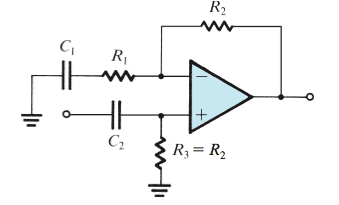# Ac coupling and offset voltage in amplifier

Hey all,

Just a question concerning the image I have provided. If I have an offset of 0.1 mV at the non inverting input, how exactly does coupling take effect in this set up? If I input an ac signal, it will pass through the cap, but will the op-amp not still experience the offset voltage?meBigGuy
Gold Member
Ignoring input bias current for the moment, a 0.1V offset at the - input means there is a 0.1mv output. That dc offset will always be there. A signal input through C2 will have that DC signal imposed on it.

Think of it this way. The opamp will output whatever is required to maintain 0.1V between + and -. Input bias currents cause a voltage drop across R2, but the effect is the same.

Is it not possible to utilize bypass capacitors such that the offset does not offset the input ac signal?

analogdesign
No bypass caps don't work that way. meBigGuy is right. Another way to think of it is you have a 0.1mV DC voltage source in front of one of your op amp inputs. How is a bypass cap going to do anything about that?

That's what I figured. There is an example in my book, however, that neglects the offset voltage that is inherent to that particular op-amp. For example, they had a nominal gain of 1000, an offset voltage of 0.03 V, and they asked the maximum input signal that would result in an ouput of 10V peak amplitude. They answered with 0.01V, which does not accout for the dc offset. I found this odd considering the dc offset was given at the beginning of the example. Just thought I would ask in case I was missing something. Thanks.

analogdesign
No worries. Offsets typically set the real-world limit to the gain you can get out of a closed-loop voltage amplifier. You can do things to cancel them but that adds complexity, of course.

Perfect, thanks again!

NascentOxygen
Staff Emeritus
For example, they had a nominal gain of 1000, an offset voltage of 0.03 V, and they asked the maximum input signal that would result in an ouput of 10V peak amplitude. They answered with 0.01V, which does not accout for the dc offset.
Another question regarding the output voltage due to bias currents for the integrator with feedback resistor $R_f$: my textbook states that the output voltage due to the bias currents is $v_o=R_fI_{OS}$, however, if non-inverting input is grounded, the only current contributing to the output voltage is the bias current entering the inverting node. Hence, $v_o=R_fI_{B^-}$. What exactly am I missing here?